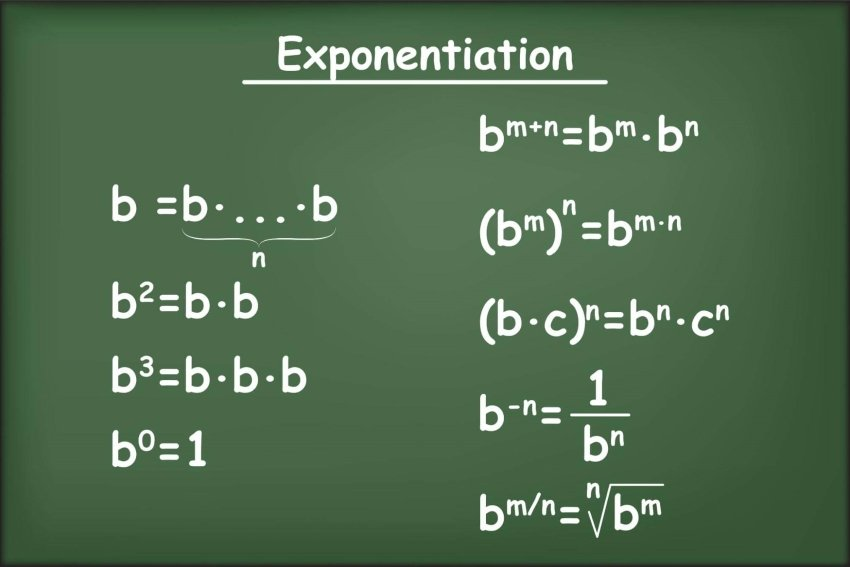View all Grade 11 Mathematics Resources

Exponents, often known as powers, are numbers that indicate how many times a base number may be multiplied by itself. For instance, the number 43 instructs you to multiply four by itself three times. The base is the number being raised by a power, whereas the exponent or power is the superscript number above it.

For example, 53 = 5 × 5 × 5 = 125; the equation is written as “five to the power of three.” The power of two is also known as “squared,” whereas the power of three is known as “cubed.” When calculating the area or volume of various forms, these words are frequently employed.

## Seven Laws of Exponents

1. Multiplying Powers with same Base.
2. Dividing Powers with the same Base.
3. Power of a Power.
4. Multiplying Powers with the same Exponents.
5. Negative Exponents.
6. Power with Exponent Zero.
7. Fractional Exponent.

## Exponent Laws Worksheets for Grade 11

Sources:

https://www.math-only-math.com/laws-of-exponents.html